## 23 January 2014

Triangle:

triangle is a polygon with three vertices, and three sides or edges that are line segments. A triangle with vertices AB, and C is denoted as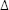ABC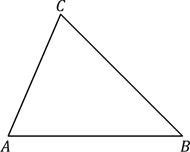The perimeter of a triangle is the sum of the lengths of its sides. If the three sides are a, b, and c, then perimeter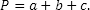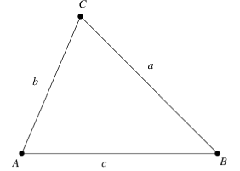The area of a triangle is the space enclosed by its three sides. It is given by the formula,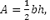where b is the base and h is the altitude.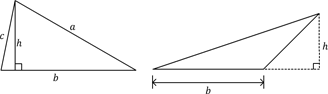simple closed figure bounded by four line segments is called a quadrilateral.
• Rectangle
• Square
• Parallelogram
• Rhombus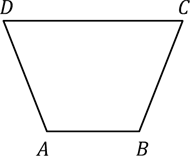Rectangle
rectangle is a quadrilateral with opposite sides equal, and each angle of measure 90o.
The perimeter of a rectangle is twice the sum of the lengths of its adjacent sides.
In the figure, the perimeter of rectangle ABCD = 2(AB + BC).
The area of a rectangle is the product of its length and breadth.
In the figure, the area of rectangle ABCD = AB x BC.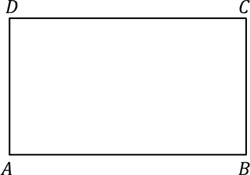Square
square is a quadrilateral with four equal sides, and each angle of measure 90o.
The perimeter of a square with side s units is 4s.
In the figure, the perimeter of square ABCD = 4AB or 4BC or 4CD or 4DA.
The area of a square with side s is s2
In the figure, the perimeter of square ABCD = AB2 or BC2 or CD2 or DA2.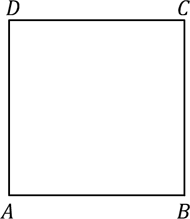Parallelogram
quadrilateral in which both the pairs of opposite sides are parallel is called a parallelogram.
The perimeter of a parallelogram is twice the sum of the lengths of the adjacent sides.
In the figure, the perimeter of parallelogram ABCD = 2(AB + BC)
The area of a parallelogram is the product of its base and perpendicular height or altitude.
Any side of a parallelogram can be taken as the base. The perpendicular dropped on that side from the opposite vertex is known as the height (altitude).
In the figure, the area of parallelogram ABCD = AB x DE or AD x BF.
Rhombus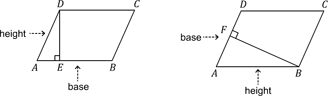parallelogram in which the adjacent sides are equal is called a rhombus.
The perimeter and area of a rhombus can be calculated using the same formula as that for a parallelogram.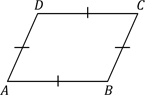#### Circle:

A circle is defined as a collection of points on a plane that are at an equal distance from a fixed point on the plane.  The fixed point is called the centre of the circle.

#### Circumference:

The distance around a circular region is known as its circumference.

#### Diameter:

Any straight line segment that passes through the centre of a circle and whose end points are on the circle is called its diameter.

Any line segment from the centre of the circle to its circumference.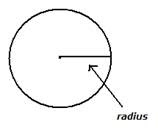Circumference of a circle =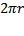, where r is the radius of the circle  or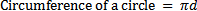, where d is the diameter of the circle.
Circumference = Diameter x 3.14
Diameter(d) is equal to twice radius(r).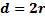Circles with the same centre but different radius are called concentric circles.

#### Circle:

The area of a circle is the region enclosed in the circle.
The area of a circle can be calculated by using the formula:
•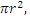if radius r is given
•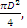if diameter D is given
•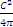if circumference C is given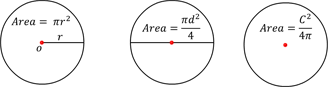With our free PDF notes you can get success in any competitive or entrance exams like CTET,  KVS, NET, CAT, MAT, CMAT, SSC, B.ED, IBPS Recruitment, IAS, CSAT, State Civil Services Exams, UPTET, PSTET, HTET & many more. It also provides NCERT solutions, CBSE, NTSE, Olympiad study material, Indian General Knowledge, English, Hindi, Mathematics, Current affairs, Science, S.ST, model test papers, important Questions and Answers asked in CBSE examinations.